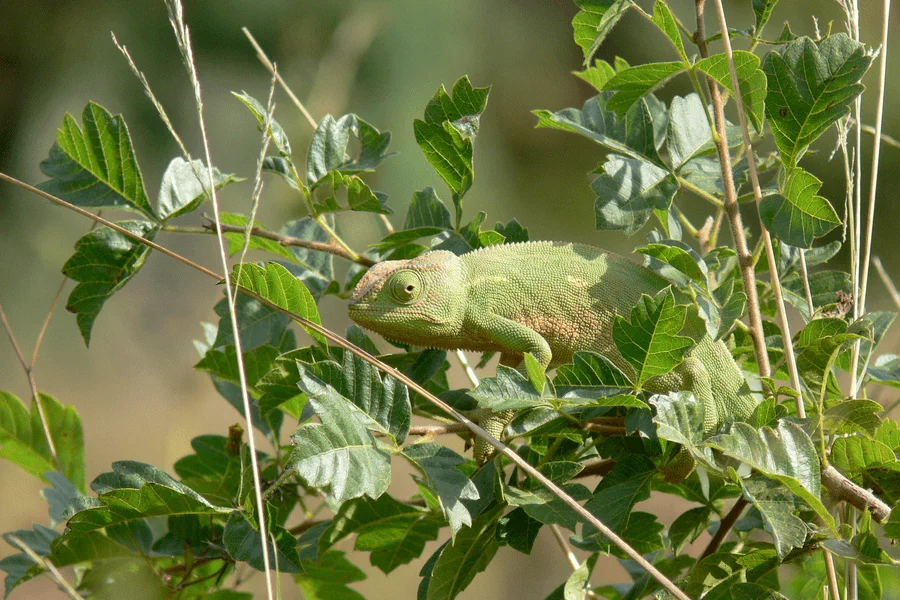# Fool the Machine

Trick neural network classifiers

By Rafael Ballestas | August 13, 2019 | Category: Development

Artificial Neural Networks (`ANNs`) are certainly a wondrous achievement. They solve classification and other learning tasks with great accuracy. However, they are not flawless and might misclassify certain inputs. No problem, some error is expected. But what if you could give it two inputs that are virtually identical, but you get different outputs? Worse, what if one is correctly classified but the other has been manipulated so that it is classified as anything you want? Could these adversarial examples be the bane of neural networks?

That is what happened with one PicoCTF challenge we came across recently. There is an application whose sole purpose is to accept a user-uploaded image, classify it, and let you know the results. Our task was to take the image of a dog, correctly classified as a Malinois, and manipulate it so that it is classified as a tree frog. However, for your image to be a proper adversarial example, it must be perceptually indistinguishable from the original, in other words, it must still look like the same previously-classified dog to a human.Figure 1. Challenge description.

The applications are potentially endless. You could:

• fool image recognition systems like physical security cameras, as does this Stealth T-shirt.• make an autonomous car crash.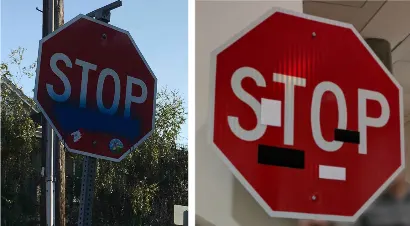• confuse virtual assistants.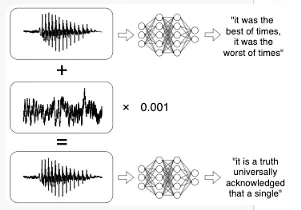• bypass spam filters, etc.

So, how does one go about creating such an adversarial example? Recall that in our brief survey of machine learning techniques, we discussed training neural networks. It is an iterative process in which you continuously adjust the weight parameters of your black box (the `ANN`) until the outputs agree with the expected ones, or at least, minimize the cost function, which is a measure of how wrong the prediction is. I will borrow an image that better explains it from an article by Adam Geitgey .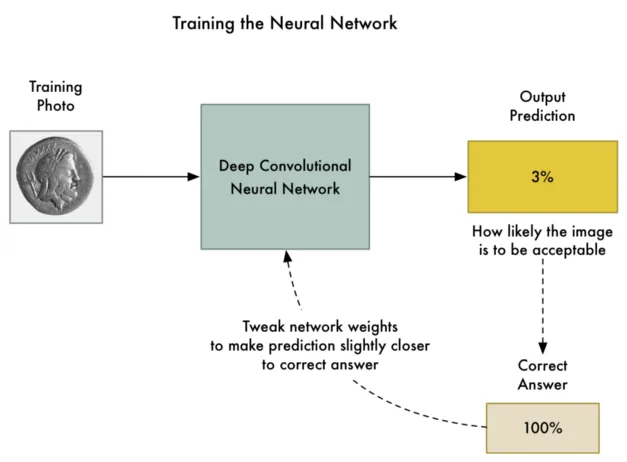Figure 2. Training a neural network by .

This technique is known as backpropagation. Now, in order to obtain a picture that is still like the original, but will classify as something entirely different, what one could do is add some noise; but not too much noise, so the picture doesn’t change, and not just anywhere, but exactly in the right places, so that the classifier reads a different pattern. Some clever folks from Google found out that the best way to do this is by using the gradient of the cost function.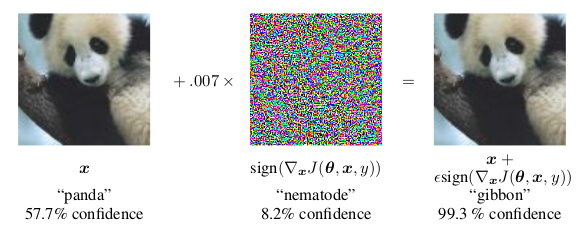Figure 3. Adding noise to fool the classifier. From 

This is called the fast gradient sign method. This gradient can be computed using backpropagation but in reverse. Since the model is already trained, and we can’t modify it, let’s modify the picture little by little and see if it gets us any closer to the target. I will again borrow from `@ageitgey` since the analogy is much clearer this way.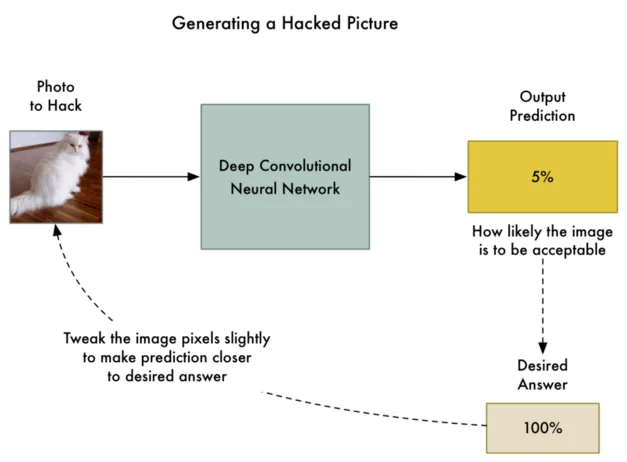Figure 4. Tweaking the image, by .

The pseudo-code that would generate an adversarial example via this method would be as follows. Assume that the model is saved in a `Keras` `h5` file, as in the challenge. `Keras` is a popular high-level neural networks `API` for `Python`. We can load the model, get the input and output layers (first and last), get the cost and gradient functions and define a convenience function that returns both for a particular input, like this:

Getting cost function and gradients from a neural network.

``````from keras.models import load_model
from keras import backend as K

input_layer            = model.layers.input
output_layer           = model.layers[-1].output
cost_function          = output_layer[0, object_type_to_fake]
get_cost_and_gradients = K.function([input_layer, K.learning_phase()],
``````

Where `object_type_to_fake` is the class number of what we want to fake. Now, according to the formula in figure 3 above, we should add a small fraction of the sign of the gradient, until we achieve the result. The result should be that the confidence in the prediction becomes at least 95%.

``````while confidence < 0.95:
``````

However, this procedure takes way too long without a `GPU`. A few hours according to Geitgey . For the `CTFer` and the more practical-minded reader, there is a library that does this and other attacks on machine learning systems to determine their vulnerability to adversarial examples: CleverHans. Using this library, we change the expensive `while` cycle above to two `API` calls: make an instance of the attack method and then ask it to generate the adversarial example.

``````from cleverhans.attacks import MomentumIterativeMethod

method = MomentumIterativeMethod(model, sess=K.get_session())
test   = method.generate_np(adversarial_image, eps=0.3, eps_iter=0.06,
nb_iter=10, y_target=target)
``````

In this case, we used a different attack, namely the `MomentumIterativeMethod` because, in this situation, it gives better results than the `FastGradientMethod`, obviously also a part of `CleverHans`. And so we obtain our adversarial example.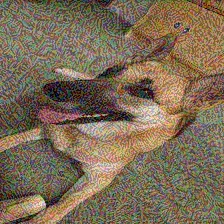Figure 5. Adversarial image for the challenge

You can almost see the tree frog lurking in the back, if you imagine the two knobs on the cabinet are its eyes. Just kidding. Upload it to the challenge site and, instead of getting the predictions, we get the flag.

Not just that, the model which is based on `MobileNet`, is 99.99974% certain that this is a tree frog. However, the difference between it and the original image, according to the widely used perceptual hash algorithm, is less than two bits. Still, the adversarial example has artifacts, at least to a human observer.

What is worse is that these issues persist across different models as long as the training data is similar. That means that we could probably pass the same image to a different animal image classifier and still get the same results.

Ultimately, we should think twice before deploying `ML`-powered security measures. This is, of course, a mock example, but in more critical situations, having models that are not resistant to adversarial examples could result in catastrophic effects. Apparently, the reason behind this is the linearity within the functions hidden in these networks. So switching to a more non-linear model, such as RBF networks, could solve the problem. Another workaround could be to train the `ANNs` including adversarial examples.

To borrow a phrase from carpenters, "Measure twice, cut once." We should also remember that whatever the solution, it should be clear that one should test twice, and deploy once.

## References

1. I. Goodfellow, J. Shlens, C. Szegedy. EXPLAINING AND HARNESSING ADVERSARIAL EXAMPLES. arXiv.

2. A. Geigtey. Machine Learning is Fun Part 8: How to Intentionally Trick Neural Networks. Medium

Ready to try Continuous Hacking?

Discover the benefits of our comprehensive Continuous Hacking solution, which hundreds of organizations are already enjoying.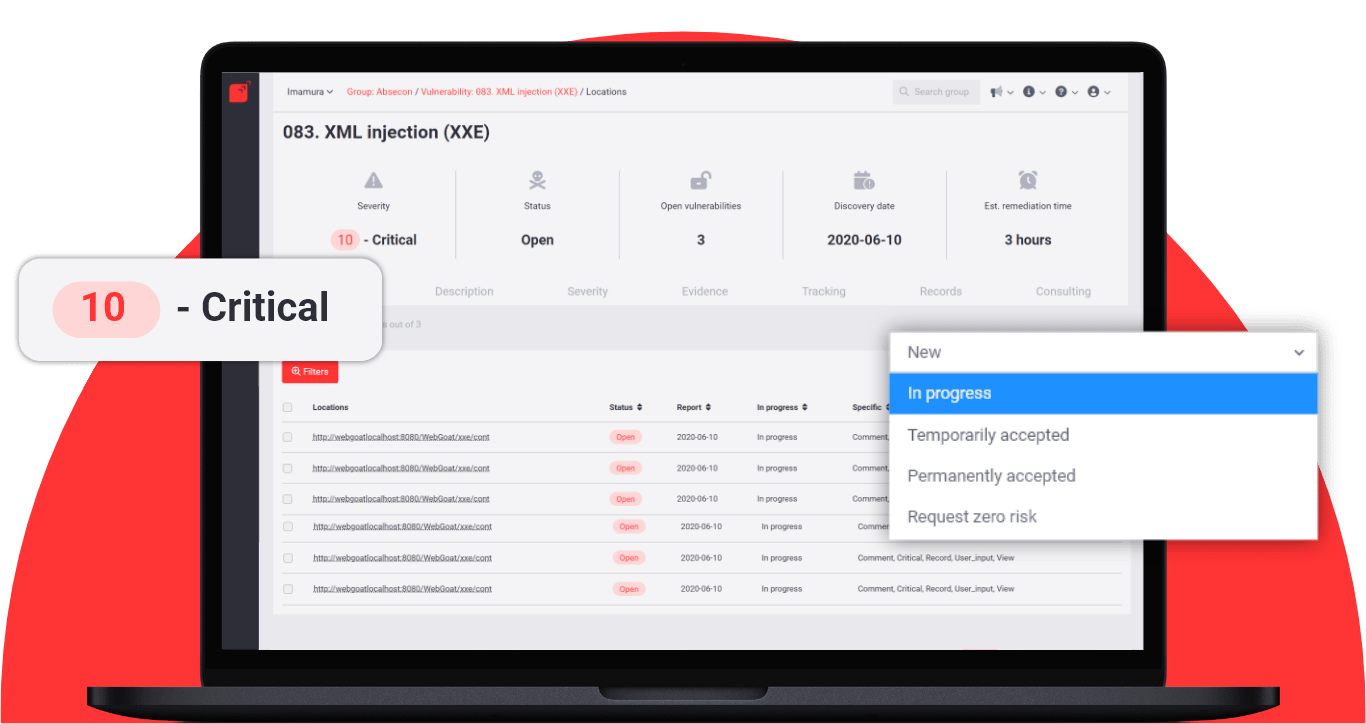Start free trial